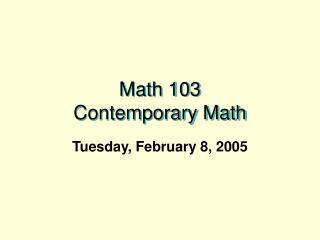# Math 103 Contemporary Math - PowerPoint PPT PresentationDownload PresentationMath 103 Contemporary Math

Math 103 Contemporary Math
Download Presentation## Math 103 Contemporary Math

- - - - - - - - - - - - - - - - - - - - - - - - - - - E N D - - - - - - - - - - - - - - - - - - - - - - - - - - -
##### Presentation Transcript

1. Math 103 Contemporary Math Tuesday, February 8, 2005

2. Review from last class FAPP video on Tilings of the plane.

3. Symmetry Ideas Reflective symmetry: BI LATERAL SYMMETRY T  C  O   0    I   A  • Folding line: "axis of symmetry" • The "flip.“ • The "mirror."

4. R(P) = P': A Transformation Before: P .... After : P' If P is on the line (axis), then R(P)=P. "P remains fixed by the reflection." If P is not on the axis, then the line PP' is perpendicular to the axis and if Q is the point of intersection of PP' with the axis then m(PQ) = m(P'Q).

5. Definition • We say F has a reflective symmetry wrt a line lif  there is a reflection  R about the line l where  R(P)=P' is still an element of Ffor every P in F.... • i.e.. R (F) = F. • l is called the axis of symmetry. • Examples of reflective symmetry:Squares...  People

6. Rotational Symmetry • Center of rotation. "rotational pole" (usually O) and angle/direction of rotation. • The "spin.“

7. R(P) = P' : A transformation • If O is the center then R(O) = O. • If the angle is 360 then R(P) = P for all P.... called the identity transformation. • If the angle is between 0 and 360 then only the center remains fixed. • For any point P the angle POP'  is the same. • Examples of rotational symmetry.

8. Single Figure Symmetries • Now... what about finding all the reflective and rotational symmetries of a single figure? • Symmetries of playing card.... • Classify the cards having the same symmetries. Notice symmetry of clubs, diamonds, hearts, spades.  • Organization of markers.

9. Symmetries of an equilateral triangle

10. Why are there only six? • Before: AAfter : A  or    B  or     CSuppose I know where A goes:What about B?  If A -> A     Before: B   After: B or C                          If  A ->B     Before:B    After: A or C                          If  A ->C     Before: B   After: A or BBy an analysis of a "tree" we count there are exactly and only 6 possibilities for where the vertices can be transformed.

11. Tree Analysis Identity B C A Reflection C B C Reflection A B A Rotation C B Rotation A C Reflection A B

12. What about combining transformations to give new symmetries Think of a symmetry as a transformation: Example: V will mean reflection across the line that is the vertical altitude of the equilateral triangle.Then let's consider a second symmetry, R=R120, which will rotate the equilateral triangle counterclockwise about its center O by 120 degrees. We now can think of first performing V to the figure and then performing R to the figure.   We will denote this V*R... meaning V followed by R.[Note that order can make a difference here, and there is an alternative  convention for this notation that would reverse the order and say that R*V means V followed by R.]Does the resulting transformation V*R also leave the equilateral  covering the same position in which it started?

13. Symmetry “Products” • V*R     =   ? • If so it is also a symmetry.... which of the six is it? • What about other products?  • This gives a  "product" for symmetries.If S and R are any symmetries of a figure then S*R is also a symmetry of the figure.

14. A "multiplication" table for Symmetries

15. Activity • Do Activity. • This shows that R240*V = ? • This "multiplicative" structure  is called the Group of symmetries of the equilateral triangle.Given any figure we can talk about the group of its symmetries.Does a figure always have at least one symmetry? .....Yes... The Identity symmetry.Such a symmetry is called the trivial symmetry.So we can compare objects for symmetries.... how many?Does the multiplication table for the symmetries look the same in some sense?